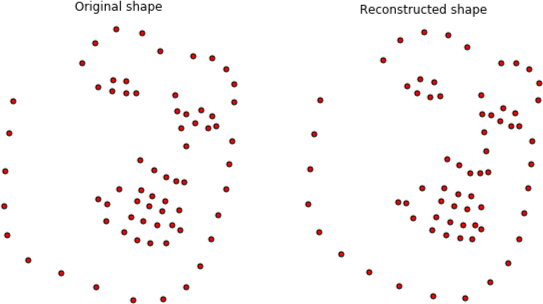# Point Distribution ModelComparing the original and reconstructed shapes after projecting into the PDM space. credit: menpofit

A Point Distribution Model (PDM) is a statistical parametric model of the shape of a deformable object. The PDN is often used to parameterize the shape of a collection of keypoints for use in deformable model, such as the Active Appearance Model (AAM). The PDM is also an efficient parameterization for keypoint filtering to remove jitter and outliers.

## Constructing the PDM

Given a set of 2D keypoints, $(x_i, y_i) \forall i = 1,...,L$, we can flatten the coordinates into a ‘shape vector’ of size $2L \times 1$:

Given a large collection of shape vectors, we can construct a PDM offline using the following algorithm:

1. Compute the global similarity transform matrix using Generalized Procrustes Analysis given the N shape vectors. Apply the global transform to the shapes, effectively normalizing scaling, in-plane rotation, and translation across the shapes.
2. Apply Principle Component Analysis (PCA) on the aligned shapes to obtain a basis of eigenvectors $U_s\in{\mathbb{R}^{N \times 2L}}$.
3. Re-orthonormalize the PCA eigenvectors given the global similarity transform basis.

The final PDM will be described by:

• $A_s\in{\mathbb{R}^{4\times2L}}$ similarity model
• $\bar{a}_s\in{\mathbb{R}^{2L}}$ similarity mean shape
• $U_s\in{\mathbb{R}^{n \times 2L}}$ PCA shape components
• $\bar{u}_s\in{\mathbb{R}^{2L}}$ PCA mean shape
• $u_e\in{\mathbb{R}^n}$ PCA eigenvalues where $n$ identifies the first $n$ eigenvectors in the PCA shape basis.

## Applying the PDM

Given a shape vector $s$, the PDM weights $p$ can be recovered by projecting into the PCA space $p = U^T_s(s - \bar{s})$. However, before projecting into the PCA space the shape must be rescaled and aligned with the mean shape $\bar{a}_s$ by applying Procrustes Analysis.

• C++
• Eigen
• Python